Updating search results...

# 121 Results

View
Selected filters:
• equations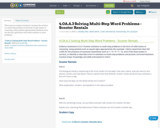Conditional Remix & Share Permitted
CC BY-NC-SA
Rating
0.0 stars

This resource requires students to present the solution to a multi-step problem in the form of valid chains of reasoning, using symbols appropriately. Students must use the four operations with whole numbers to solve problems.

Subject:
Education
Mathematics
Material Type:
Activity/Lab
Assessment
Homework/Assignment
Lesson
10/04/2018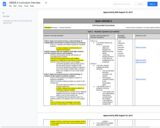Conditional Remix & Share Permitted
CC BY-NC-SA
Rating
0.0 stars

6th Grade Regular Math: Course Scope & Sequence.

Subject:
Mathematics
Material Type:
Full Course
Provider:
Liberty Public Schools
08/15/2017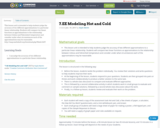Conditional Remix & Share Permitted
CC BY-NC
Rating
0.0 stars

This lesson unit is intended to help students judge the accuracy of two different approximations to a particular linear relationship. Students will compare two linear functions as approximations to the relationship between Celsius and Fahrenheit temperature and consider under what circumstances each of the approximations may be reasonable.

Subject:
Mathematics
Material Type:
Lesson
01/19/2017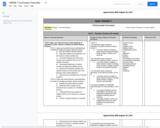Conditional Remix & Share Permitted
CC BY-NC-SA
Rating
0.0 stars

7th Grade Regular Math: Course Scope & Sequence.

Subject:
Mathematics
Material Type:
Full Course
Provider:
Liberty Public Schools
08/15/2017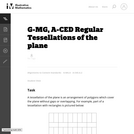Unrestricted Use
CC BY
Rating
0.0 stars

This task examines the ways in which the plane can be covered by regular polygons in a very strict arrangement called a regular tessellation. These tessellations are studied here using algebra, which enters the picture via the formula for the measure of the interior angles of a regular polygon (which should therefore be introduced or reviewed before beginning the task). The goal of the task is to use algebra in order to understand which tessellations of the plane with regular polygons are possible.

Subject:
Algebra
Geometry
Mathematics
Material Type:
Activity/Lab
Provider:
Illustrative Mathematics
Provider Set:
Illustrative Mathematics
Author:
Illustrative Mathematics
01/21/2013Unrestricted Use
CC BY
Rating
0.0 stars

This task provides a simple but interesting and realistic context in which students are led to set up a rational equation (and a rational inequality) in one variable, and then solve that equation/inequality for an unknown variable.

Subject:
Algebra
Mathematics
Material Type:
Activity/Lab
Provider:
Illustrative Mathematics
Provider Set:
Illustrative Mathematics
Author:
Illustrative Mathematics
12/15/2012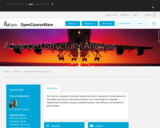Conditional Remix & Share Permitted
CC BY-NC-SA
Rating
0.0 stars

This course is designed to introduce students who wish to specialize in stress analysis of thin-walled structures to more advanced topics such as the analysis of statically indeterminate structures, warping, constraint stresses, shear diffusion, and elements of plate bending.

Subject:
Applied Science
Engineering
Material Type:
Full Course
Provider:
Delft University of Technology
Provider Set:
Delft University OpenCourseWare
Author:
Dr. Mostafa Abdalla
02/07/2014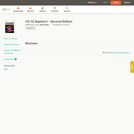Conditional Remix & Share Permitted
CC BY-NC-SA
Rating
0.0 stars

A work in progress, CK-12's Algebra I Second Edition is a clear presentation of algebra for the high school student. Topics include: Equations and Functions, Real Numbers, Equations of Lines, Solving Systems of Equations and Quadratic Equations.

Subject:
Algebra
Functions
Mathematics
Material Type:
Textbook
Provider:
CK-12 Foundation
Provider Set:
CK-12 FlexBook
Author:
Andrew
Annamaria
Anne
Eve
Farbizio
Gloag
Rawley
12/03/2010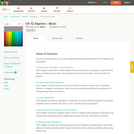Conditional Remix & Share Permitted
CC BY-NC-SA
Rating
0.0 stars

CK-12 Foundation's Basic Algebra FlexBook is an introduction to the algebraic topics of functions, equations, and graphs for middle-school and high-school students.

Subject:
Algebra
Functions
Mathematics
Material Type:
Textbook
Provider:
CK-12 Foundation
Provider Set:
CK-12 FlexBook
Author:
Farbizio, Annamaria
Gloag, Andrew
Gloag, Anne
Kramer, Melissa
09/21/2010Conditional Remix & Share Permitted
CC BY-NC-SA
Rating
0.0 stars

This site teaches High Schoolers how to create equations through a series of 298 questions and interactive activities aligned to 5 Common Core mathematics skills.

Subject:
Algebra
Mathematics
Material Type:
Activity/Lab
Interactive
Provider:
Provider Set:
01/09/2015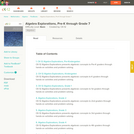Conditional Remix & Share Permitted
CC BY-NC-SA
Rating
0.0 stars

CK-12 Algebra Explorations is a hands-on series of activities that guides students from Pre-K to Grade 7 through algebraic concepts.

Subject:
Algebra
Mathematics
Material Type:
Activity/Lab
Teaching/Learning Strategy
Provider:
CK-12 Foundation
Provider Set:
CK-12 FlexBook
Author:
Carol ­ Findell, Mary ­Cavanagh, Carole ­Greenes
02/23/2012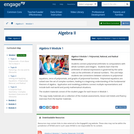Conditional Remix & Share Permitted
CC BY-NC-SA
Rating
0.0 stars

"Students connect polynomial arithmetic to computations with whole numbers and integers.  Students learn that the arithmetic of rational expressions is governed by the same rules as the arithmetic of rational numbers.  This unit helps students see connections between solutions to polynomial equations, zeros of polynomials, and graphs of polynomial functions.  Polynomial equations are solved over the set of complex numbers, leading to a beginning understanding of the fundamental theorem of algebra.  Application and modeling problems connect multiple representations and include both real world and purely mathematical situations.

Find the rest of the EngageNY Mathematics resources at https://archive.org/details/engageny-mathematics.

Subject:
Algebra
Mathematics
Material Type:
Module
Provider:
New York State Education Department
Provider Set:
EngageNY
05/14/2013Conditional Remix & Share Permitted
CC BY-NC-SA
Rating
0.0 stars

(Nota: Esta es una traducción de un recurso educativo abierto creado por el Departamento de Educación del Estado de Nueva York (NYSED) como parte del proyecto "EngageNY" en 2013. Aunque el recurso real fue traducido por personas, la siguiente descripción se tradujo del inglés original usando Google Translate para ayudar a los usuarios potenciales a decidir si se adapta a sus necesidades y puede contener errores gramaticales o lingüísticos. La descripción original en inglés también se proporciona a continuación.)

"Los estudiantes conectan la aritmética polinomial con los cálculos con números enteros e enteros. Los estudiantes aprenden que la aritmética de las expresiones racionales se rige por las mismas reglas que la aritmética de los números racionales. Esta unidad ayuda a los estudiantes a ver conexiones entre soluciones a ecuaciones polinomiales, ceros de polinomiales,, y gráficos de funciones polinómicas. Las ecuaciones polinomiales se resuelven sobre el conjunto de números complejos, lo que lleva a una comprensión inicial del teorema fundamental del álgebra. Los problemas de aplicación y modelado conectan múltiples representaciones e incluyen situaciones de mundo real y puramente matemáticas.

Encuentre el resto de los recursos matemáticos de Engageny en https://archive.org/details/engageny-mathematics.

English Description:
"Students connect polynomial arithmetic to computations with whole numbers and integers.  Students learn that the arithmetic of rational expressions is governed by the same rules as the arithmetic of rational numbers.  This unit helps students see connections between solutions to polynomial equations, zeros of polynomials, and graphs of polynomial functions.  Polynomial equations are solved over the set of complex numbers, leading to a beginning understanding of the fundamental theorem of algebra.  Application and modeling problems connect multiple representations and include both real world and purely mathematical situations.

Find the rest of the EngageNY Mathematics resources at https://archive.org/details/engageny-mathematics.

Subject:
Algebra
Mathematics
Material Type:
Module
Provider:
New York State Education Department
Provider Set:
EngageNY
05/14/2013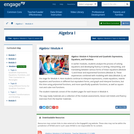Conditional Remix & Share Permitted
CC BY-NC-SA
Rating
0.0 stars

In earlier modules, students analyze the process of solving equations and developing fluency in writing, interpreting, and translating between various forms of linear equations (Module 1) and linear and exponential functions (Module 3). These experiences combined with modeling with data (Module 2), set the stage for Module 4. Here students continue to interpret expressions, create equations, rewrite equations and functions in different but equivalent forms, and graph and interpret functions, but this time using polynomial functions, and more specifically quadratic functions, as well as square root and cube root functions.

Find the rest of the EngageNY Mathematics resources at https://archive.org/details/engageny-mathematics.

Subject:
Algebra
Mathematics
Material Type:
Module
Provider:
New York State Education Department
Provider Set:
EngageNY
09/17/2013Conditional Remix & Share Permitted
CC BY-NC-SA
Rating
0.0 stars

(Nota: Esta es una traducción de un recurso educativo abierto creado por el Departamento de Educación del Estado de Nueva York (NYSED) como parte del proyecto "EngageNY" en 2013. Aunque el recurso real fue traducido por personas, la siguiente descripción se tradujo del inglés original usando Google Translate para ayudar a los usuarios potenciales a decidir si se adapta a sus necesidades y puede contener errores gramaticales o lingüísticos. La descripción original en inglés también se proporciona a continuación.)

En módulos anteriores, los estudiantes analizan el proceso de resolver ecuaciones y desarrollar fluidez en la escritura, interpretación y traducción entre varias formas de ecuaciones lineales (Módulo 1) y funciones lineales y exponenciales (Módulo 3). Estas experiencias combinadas con el modelado con datos (Módulo 2), preparan el escenario para el módulo 4. Aquí los estudiantes continúan interpretando expresiones, crean ecuaciones, reescriben ecuaciones y funciones en formas diferentes pero equivalentes, y gráficos e interpretan funciones, pero esta vez utilizando polinomial funciones y funciones más específicamente cuadráticas, así como funciones de raíz de raíz cuadrada y de cubos.

Encuentre el resto de los recursos matemáticos de Engageny en https://archive.org/details/engageny-mathematics.

English Description:
In earlier modules, students analyze the process of solving equations and developing fluency in writing, interpreting, and translating between various forms of linear equations (Module 1) and linear and exponential functions (Module 3). These experiences combined with modeling with data (Module 2), set the stage for Module 4. Here students continue to interpret expressions, create equations, rewrite equations and functions in different but equivalent forms, and graph and interpret functions, but this time using polynomial functions, and more specifically quadratic functions, as well as square root and cube root functions.

Find the rest of the EngageNY Mathematics resources at https://archive.org/details/engageny-mathematics.

Subject:
Algebra
Mathematics
Material Type:
Module
Provider:
New York State Education Department
Provider Set:
EngageNY
09/17/2013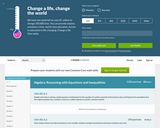Conditional Remix & Share Permitted
CC BY-NC-SA
Rating
0.0 stars

This site teaches Reasoning with Equations and Inequalities to High Schoolers through a series of 5909 questions and interactive activities aligned to 36 Common Core mathematics skills.

Subject:
Algebra
Mathematics
Material Type:
Activity/Lab
Interactive
Provider:
Provider Set:
01/09/2015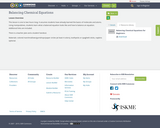Conditional Remix & Share Permitted
CC BY-NC
Rating
0.0 stars

This lesson is one to two hours long. It assumes students have already learned the basics of molecules and atoms. Using manipulatives, students learn what a balanced equation looks like and how to balance an equation. Additional links are included.There is a teacher plan and a student handout.Materials: colored marshmallows/gumdrops/paper circles (at least 4 colors), toothpicks or spaghetti sticks, napkins optional.

Subject:
Applied Science
Material Type:
Lesson Plan
Author:
Shana Friend
03/26/2019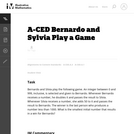Unrestricted Use
CC BY
Rating
0.0 stars

This task presents a simple but mathematically interesting game whose solution is a challenging exercise in creating and reasoning with algebraic inequalities. The core of the task involves converting a verbal statement into a mathematical inequality in a context in which the inequality is not obviously presented, and then repeatedly using the inequality to deduce information about the structure of the game.

Subject:
Algebra
Mathematics
Material Type:
Activity/Lab
Provider:
Illustrative Mathematics
Provider Set:
Illustrative Mathematics
Author:
Illustrative Mathematics
10/21/2013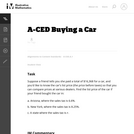Unrestricted Use
CC BY
Rating
0.0 stars

The emphasis in this task is on the progression of equations, from two that involve different values of the sales tax, to one that involves the sales tax as a parameter. It is designed to foster the habit of looking for regularity in solution procedures, so that students don't approach every equation as a new problem but learn to notice familiar types.

Subject:
Algebra
Mathematics
Material Type:
Activity/Lab
Provider:
Illustrative Mathematics
Provider Set:
Illustrative Mathematics
Author:
Illustrative Mathematics
05/01/2012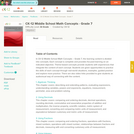Conditional Remix & Share Permitted
CC BY-NC-SA
Rating
0.0 stars

CK-12 Middle School Math Concepts for seventh grade provides a complete textbook. It presents topics including algebraic thinking, patterns, decimals, decimal operations, fractions, fraction operations, integers, integer operations, ratios, rates, proportions, percents, percent applications, equations, solving equations, inequalities, functions, graphing functions, geometry, plane geometry, solid geometry, area, perimeter, surface area, volume, statistics including mean, median, mode and range, graphing and types of graphs, and probability.

Subject:
Algebra
Geometry
Mathematics
Numbers and Operations
Statistics and Probability
Material Type:
Textbook
Provider:
CK-12 Foundation
Provider Set:
CK-12 FlexBook
Author:
Jen Kershaw